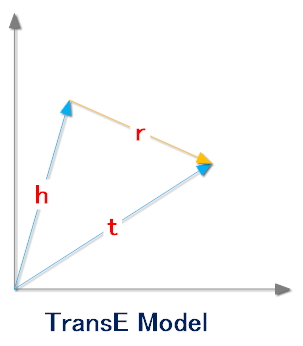# 知识表示-TransE

Posted by Sunder on July 7, 2016

## 预备知识

（姚明，出生于，上海）

（姚明，职业，篮球运动员）

（姚明，性别，男）

• h表示头实体
• r表示关系
• t表示尾实体

• $$h$$ 表示头实体对应的向量
• $$r$$ 表示关系对应的向量
• $$t$$ 表示尾实体对应的向量

[0.01, 0.04, 0.8, 0.32, 0.09, 0.18]

## TransE的基本思想

TransE模型认为一个正确的三元组的embedding$$(h,r,t)$$会满足下面的公式:$$h+r=t$$## TransE的目标函数

$min\sum_{(h,r,t)\in\Delta}\sum_{(h',r',t')\in\Delta'}[\gamma+f(h,r,t)-f(h',r',t')]_+$

• $$\Delta$$ 表示正确的三元组集合
• $$\Delta'$$ 表示错误的三元组集合
• $$\gamma$$ 表示正负样本之间的间距，是一个常数
• $$[x]_+$$ 表示$$max(0,x)$$

## 负样本（错误三元组）的产生

（姚明，出生于，上海）

（姚明，出生于，上海）

（姚明，出生于，男）

transE的主要内容就是这么多了，后续可能会添加如何编程实现transE的内容。 如何用TensorFlow实现transE可以看这里